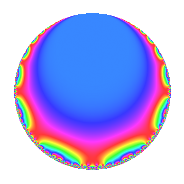Properties

 Label 8016.2.a.eLevel 8016 Weight 2 Character orbit 8016.a Self dual Yes Analytic conductor 64.008 Analytic rank 1 Dimension 1 CM No Inner twists 1

Related objects

Newspace parameters

 Level: $$N$$ = $$8016 = 2^{4} \cdot 3 \cdot 167$$ Weight: $$k$$ = $$2$$ Character orbit: $$[\chi]$$ = 8016.a (trivial)

Newform invariants

 Self dual: Yes Analytic conductor: $$64.0080822603$$ Analytic rank: $$1$$ Dimension: $$1$$ Coefficient field: $$\mathbb{Q}$$ Coefficient ring: $$\mathbb{Z}$$ Coefficient ring index: $$1$$ Fricke sign: $$1$$ Sato-Tate group: $\mathrm{SU}(2)$

$q$-expansion

 $$f(q)$$ $$=$$ $$q$$ $$\mathstrut -\mathstrut q^{3}$$ $$\mathstrut +\mathstrut q^{9}$$ $$\mathstrut +\mathstrut O(q^{10})$$ $$q$$ $$\mathstrut -\mathstrut q^{3}$$ $$\mathstrut +\mathstrut q^{9}$$ $$\mathstrut +\mathstrut 2q^{17}$$ $$\mathstrut +\mathstrut 8q^{19}$$ $$\mathstrut +\mathstrut 4q^{23}$$ $$\mathstrut -\mathstrut 5q^{25}$$ $$\mathstrut -\mathstrut q^{27}$$ $$\mathstrut -\mathstrut 10q^{29}$$ $$\mathstrut -\mathstrut 8q^{31}$$ $$\mathstrut -\mathstrut 8q^{37}$$ $$\mathstrut +\mathstrut 2q^{41}$$ $$\mathstrut -\mathstrut 2q^{43}$$ $$\mathstrut -\mathstrut 7q^{49}$$ $$\mathstrut -\mathstrut 2q^{51}$$ $$\mathstrut -\mathstrut 12q^{53}$$ $$\mathstrut -\mathstrut 8q^{57}$$ $$\mathstrut +\mathstrut 6q^{59}$$ $$\mathstrut +\mathstrut 14q^{61}$$ $$\mathstrut +\mathstrut 6q^{67}$$ $$\mathstrut -\mathstrut 4q^{69}$$ $$\mathstrut -\mathstrut 12q^{71}$$ $$\mathstrut +\mathstrut 2q^{73}$$ $$\mathstrut +\mathstrut 5q^{75}$$ $$\mathstrut +\mathstrut 4q^{79}$$ $$\mathstrut +\mathstrut q^{81}$$ $$\mathstrut +\mathstrut 6q^{83}$$ $$\mathstrut +\mathstrut 10q^{87}$$ $$\mathstrut +\mathstrut 10q^{89}$$ $$\mathstrut +\mathstrut 8q^{93}$$ $$\mathstrut -\mathstrut 2q^{97}$$ $$\mathstrut +\mathstrut O(q^{100})$$

Embeddings

For each embedding $$\iota_m$$ of the coefficient field, the values $$\iota_m(a_n)$$ are shown below.

For more information on an embedded modular form you can click on its label.

Label $$\iota_m(\nu)$$ $$a_{2}$$ $$a_{3}$$ $$a_{4}$$ $$a_{5}$$ $$a_{6}$$ $$a_{7}$$ $$a_{8}$$ $$a_{9}$$ $$a_{10}$$
1.1
 0
0 −1.00000 0 0 0 0 0 1.00000 0
 $$n$$: e.g. 2-40 or 990-1000 Significant digits: Format: Complex embeddings Normalized embeddings Satake parameters Satake angles

Inner twists

This newform does not admit any (nontrivial) inner twists.

Atkin-Lehner signs

$$p$$ Sign
$$2$$ $$1$$
$$3$$ $$1$$
$$167$$ $$1$$

Hecke kernels

This newform can be constructed as the intersection of the kernels of the following linear operators acting on $$S_{2}^{\mathrm{new}}(\Gamma_0(8016))$$:

 $$T_{5}$$ $$T_{7}$$ $$T_{11}$$ $$T_{13}$$